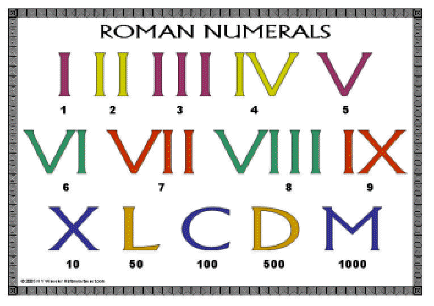КАТЕГОРИИ:

Архитектура-(3434)Астрономия-(809)Биология-(7483)Биотехнологии-(1457)Военное дело-(14632)Высокие технологии-(1363)География-(913)Геология-(1438)Государство-(451)Демография-(1065)Дом-(47672)Журналистика и СМИ-(912)Изобретательство-(14524)Иностранные языки-(4268)Информатика-(17799)Искусство-(1338)История-(13644)Компьютеры-(11121)Косметика-(55)Кулинария-(373)Культура-(8427)Лингвистика-(374)Литература-(1642)Маркетинг-(23702)Математика-(16968)Машиностроение-(1700)Медицина-(12668)Менеджмент-(24684)Механика-(15423)Науковедение-(506)Образование-(11852)Охрана труда-(3308)Педагогика-(5571)Полиграфия-(1312)Политика-(7869)Право-(5454)Приборостроение-(1369)Программирование-(2801)Производство-(97182)Промышленность-(8706)Психология-(18388)Религия-(3217)Связь-(10668)Сельское хозяйство-(299)Социология-(6455)Спорт-(42831)Строительство-(4793)Торговля-(5050)Транспорт-(2929)Туризм-(1568)Физика-(3942)Философия-(17015)Финансы-(26596)Химия-(22929)Экология-(12095)Экономика-(9961)Электроника-(8441)Электротехника-(4623)Энергетика-(12629)Юриспруденция-(1492)Ядерная техника-(1748)

# Numerals

Look at the picture and read the following Roman numerals.

NUMERALS

UNIT 142. Match the Arabic and Roman numbers. Why are Arabic numbers used in mathematics?

 a M b C c I d D e LX f L g V h XL i X j DCCC

3. Read the text and match the sentences (A-C) to the numbered spaces (1-3) in the text:

A. Positional notation is made possible by the use of a symbol for zero.

B. Such a system is inconvenient when dealing with large numbers, and as early as 3400 BC in Egypt and 3000 BC in Mesopotamia a special symbol was adopted for the number 10.

C. The symbols are usually added together.

Numerals are signs or symbols for graphic representation of numbers. The earliest forms of number notation were simply groups of straight lines, either vertical or horizontal, each line corresponding to the number 1.

The addition of this second number symbol made it possible to express the number 11 with 2 instead of 11 individual symbols and the number 99 with 18 instead of 99 individual symbols. Later numeral systems introduced extra symbols for a number between 1 and 10 and additional symbols for numbers greater than 10.

ROMAN NUMERALS. The system of number symbols created by the Romans had the merit of expressing all numbers from 1 to 1,000,000 with a total of seven symbols: I for 1, V for 5, X for 10, L for 50, C for 100, D for 500, and M for 1000.

Roman numerals are read from left to right. The symbols representing the largest quantities are placed at the left; immediately to the right of those are the symbols representing the next largest quantities, and so on.

For example, LX = 60, and MMCIII = 2103. When a numeral is smaller than the numeral to the right, however, the numeral on the left should be subtracted from the numeral on the right. For instance, XIV = 14 and IX = 9. A small bar (¯) placed over the numeral multiplies the numeral by 1000. Thus, theoretically, it is possible, by using an infinite number of bars, to express the numbers from 1 to infinity. The Roman system's one drawback, however, is that it is not suitable for rapid written calculations.

ARABIC NUMERALS. The common system of number notation in use in most parts of the world today is the Arabic system. This system was first developed by the Hindus and was in use in India in the 3rd century BC. The Hindu numeral system was probably introduced into the Arab world about the 7th or 8th century AD. The first recorded use of the system in Europe was in ad 976.

The important innovation in the Arabic system was the use of positional notation, in which individual number symbols assume different values according to their position in the written numeral.

The symbol 0 makes it possible to differentiate between 11, 101, and 1001, and all numbers can be expressed in terms of ten symbols, the numerals from 1 to 9 plus 0.

Дата добавления: 2014-11-29; Просмотров: 1011; Нарушение авторских прав?; Мы поможем в написании вашей работы!

Нам важно ваше мнение! Был ли полезен опубликованный материал? Да | Нет

 ПОИСК ПО САЙТУ: .ya-page_js_yes .ya-site-form_inited_no { display: none; }

Читайте также:
studopedia.su - Студопедия (2013 - 2022) год. Все материалы представленные на сайте исключительно с целью ознакомления читателями и не преследуют коммерческих целей или нарушение авторских прав! Последнее добавление
Генерация страницы за: 0.015 сек.